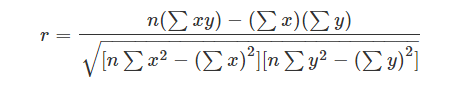# What is Correlation?

Correlation is a statistic that measures the degree to which two variables move about each other. While there are many measures of association for variables that are measured at the ordinal or higher level of measurement, It is the most commonly used approach. It is applied in quantifying the association between two continuous variables, for example, an dependent and independent variable or among two independent variables.

Correlation Coefficient

It measures to describe the extent of the statistical relationship between two interval or ratio level variables. It is scaled so that it is always between -1 and +1. When r is close to 0 this means that there is little relationship between the variables and the farther away from 0 r is, in either the positive or negative direction, the greater the relationship between the two variables.### Types of Correlation

There can be 3 such situations to see the relation between the two variables:

• Positive Correlation: When the value of one variable increases concerning another.
• Negative: When the value of one variable decreases concerning another.
• No Correlation: When there is no linear dependence or no relation between the two variables.

Correlation Formula

It shows the relation between two variables. It shows the measure of correlation. To compare two datasets, we use the correlation formulas.

Pearson Correlation Coefficient Formula

It is the most common formula used for linear dependency between the data set. The value of the coefficient lies between -1 to +1. When the coefficient comes down to zero, then the data is considered as not related. While, if we get the value of +1, then the data are positively correlated, and -1 has a negative correlation.Where n = Quantity of Information

Σx = Total of the First Variable Value

Σy = Total of the Second Variable Value

Σxy = Sum of the Product of first & Second Value

Σx2 = Sum of the Squares of the First Value

Σy2 = Sum of the Squares of the Second Value

Scatter Diagram

A scatter diagram is a diagram that shows the values of two variables X and Y, along with how these two variables relate to each other. Values of X variables are given along the horizontal axis, Ans Y variables are given on the vertical axis.